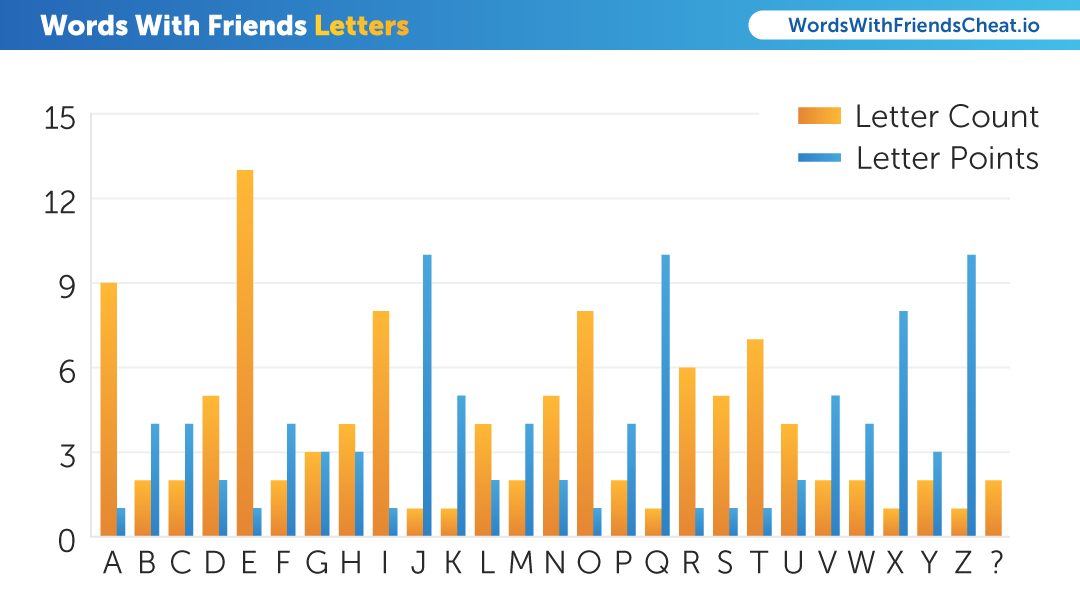# What are the Letters in Words With Friends?The letters in Words With Friends consist of 104 tiles. For each letter there are:

• A = 9 (1 point)
• B = 2 (4 points)
• C = 2 (4 points)
• D = 5 (2 points)
• E = 13 (1 point)
• F = 2 (4 points)
• G = 3 (3 points)
• H = 4 (3 points)
• I = 8 (1 points)
• J = 1 (10 points
• K = 1 (5 points)
• L = 4 (2 points)
• M = 2 (4 points)
• N = 5 (2 points)
• O = 8 (1 point)
• P = 2 (4 points)
• Q = 1 (10 points)
• R = 6 (1 point)
• S = 5 (1 point)
• T = 7 (1 point)
• U = 4 (2 points)
• V = 2 (5 points)
• W = 2 (4 points)
• X = 1 (8 points)
• Y = 2 (3 points)
• Z = 1 (10 points)
• Blank Tile = 2 (0 points)

The letters in the Words With Friends Fast Play game mode consist of 52 tiles. For each letter there are:

• A = 5 (1 point)
• B = 1 (4 points)
• C = 1 (4 points)
• D = 2 (2 points)
• E = 7 (1 point)
• F = 1 (4 points)
• G = 1 (3 points)
• H = 1 (3 points)
• I = 4 (1 points)
• J = 1 (10 points
• K = 1 (5 points)
• L = 2 (2 points)
• M = 1 (4 points)
• N = 2 (2 points)
• O = 4 (1 point)
• P = 1 (4 points)
• Q = 1 (10 points)
• R = 2 (1 point)
• S = 4 (1 point)
• T = 2 (1 point)
• U = 1 (2 points)
• V = 1 (5 points)
• W = 1 (4 points)
• X = 1 (8 points)
• Y = 1 (3 points)
• Z = 1 (10 points)
• Blank Tile = 2 (0 points)